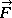# Help calculating work in an xy plane

## Homework Statement

A force= (1.9 N/m2)x2i is applied to a particle initially at rest in the xy plane. Find the work done by this force on the particle and the final speed of the particle as it moves along a path as follows. The given force is the only force doing work on the particle.

a) in a straight line from point (2.1 m, 2.1 m) to point (2.1 m, 7.5 m)
_________ J

b) in a straight line from point (2.1 m, 2.1 m) to point (4.8 m, 6.6 m)
__________ J

W = F x d

## The Attempt at a Solution

a) Due to the equation that I found above, I have used the distance formula to calculate d:
= 5.4
Then I did F x d = 1.9 * 5.4 = 10.26 (Wrong)

b) Same this as above:
= 2.7
F x d = 1.9 * 2.7 = 5.13 (Wrong)
-------------------------------------------------
On top of these incorrect attempts, I am also confused with what the problem is asking... It says to find the word done by the force, and the final speed of the particle, but the answer boxes contain a J for Joules which is a unit of work. How would I put work and speed in one box?

Last edited by a moderator:

## Answers and Replies

rude man
Homework Helper
Gold Member
Is the correct statement of the force F = 1.9 x2 i Newtons?
(where i is the unit vector in the x direction.)

PS it does not say to find the final speed. Both answers will be in Joules.

Yes that is the correct statement, sorry about that. I have corrected it.

It does say in the question "Find the work done by this force on the particle and the final speed of the particle as it moves along a path as follows", but it looks like the questions are asking for work, so I guess I'll just ignore that part.

haruspex
Science Advisor
Homework Helper
Gold Member
2020 Award
Force and displacement are vectors. What, exactly, is the formula for the work done in terms of those vectors?

Oh! It looks like it's the dot product of the force and the displacement vector.

EDIT: Okay, I got a) correct: W = F dot d = 0 + 0 = 0
Now for b) I get 5.13x2 and it is wrong. I think it might have something to do with the x

Last edited:
rude man
Homework Helper
Gold Member
Oh! It looks like it's the dot product of the force and the displacement vector.

EDIT: Okay, I got a) correct: W = F dot d = 0 + 0 = 0
Now for b) I get 5.13x2 and it is wrong. I think it might have something to do with the x

You have to integrate F dot dx to get part (b).